DECEMBER 2006

Time: 3 Hours                                                                                                     Max. Marks: 100

NOTE: There are 9 Questions in all.

·      Question 1 is compulsory and carries 20 marks. Answer to Q. 1. must be written in the space provided for it in the answer book supplied and nowhere else.

·      Out of the remaining EIGHT Questions answer any FIVE Questions. Each question carries 16 marks.

·      Any required data not explicitly given, may be suitably assumed and stated.

Q.1       Choose the correct or best alternative in the following:                                         (2x10)

a.       Euler’s method when applied to the initial value problem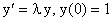, gives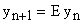. Then E is equal to

(A)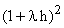.                                 (B)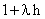.

(C)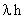.                                              (D)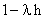.

b.      In the integral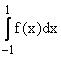, the values of the integrand are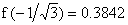, and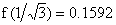. Then, the Gauss-Legendre two point formula gives

(A)    0.5434.                                        (B)  0.5406.

(C) 0.2250.                                         (D) 0.6536.

c.   The numerical differentiation formula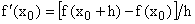is given. Then, the error is defined by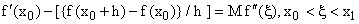The value of M is

(A)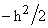.                                       (B)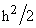.

(C)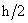.                                            (D)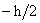.

d.    The linear least squares polynomial approximation to the following data is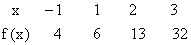(A)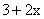(B)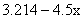(C)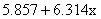.                         (D)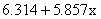.

e.   If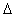is the forward difference operator, then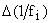is equal to

(A)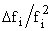.                                     (B)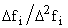.

(C)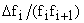.                              (D)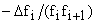.

f.    The system of equations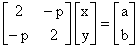is being solved by Gauss-Jacobi method. The iteration converges if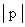is less than

(A)     2.                                                 (B)  2.5

(C)  3.0                                               (D)  4.0

g.   The iteration method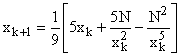converges and is determining a particular quantity. This quantity is

(A)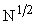.                                         (B)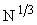.

(C)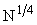.                                          (D)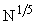.

h.   What is the output of the following C program

main()

{

int a[ ] = { 3.8, 4.4, 5, 7.6, 6 };

int i, *s = a,*t = a;

for ( i=0; i<5; i++ )

{

printf ( "% d",*a );

++t ;

}

for ( i=0; i<5; i++ )

{

printf ( "% d",*s );

++s ;

}

}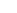(A)    3333334567.                               (B) 3333344586.

(C) 3333335576.                                (D) 3334444586.

i.    What is the output of the following C program

main()
{
int i = 2;
switch ( i )
{

default : printf ( “hundred” );

case 1: printf  ( “two hundred” );

break ;

case 2: printf  ( “five hundred” );

break ;

case 3: printf  ( “six hundred” );

break ;

}
}

(A) hundred.                                        (B)  two hundred.

(C) five hundred.                                 (D) six hundred.

j.    What is the output of the following C program

# define int char

main()
{
int i = 87;
printf ("sizeof ( i ) = %d", sizeof ( i ));
}

(A)  87                                                (B)  1

(C)  8                                                  (D) 2

Answer any FIVE Questions out of EIGHT Questions.

Each question carries 16 marks.

Q.2     a.   The smallest positive root of the equation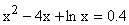is to be determined. Find an interval of unit length in which the root lies. Taking the end points of this interval as initial approximations, obtain the root correct to three decimal places using secant method.                                           (8)

b.   A method for finding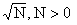is written as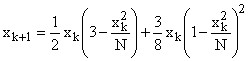Find the order of the method and the error constant.                                           (8)

Q.3     a.   Solve the system of equations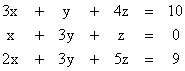using the Gauss-elimination method.     (8)

b.   The system of equations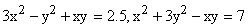has a solution near x = 1.3, y = – 1.1. Perform two iterations of the Newton’s method to improve the solution.                             (8)

Q.4     a.   The following system of equations is given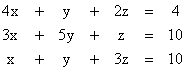.

The system is to be solved by the Gauss-Seidel iteration method. Obtain the iteration matrix of the method. Hence, find the rate of convergence of the method.                                                             (10)

b.  Find the Choleski decomposition of the symmetric matrix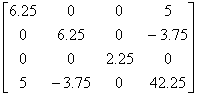(6)

Q.5      a.   An equi-spaced data with step length h is to be constructed for a function f ( x ) and approximations to function values  are to  be calculated by linear interpolation from this table. Find the maximum step size that can be used. The function values are to be correct to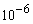for the function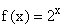over the interval [0, 1 ].                                                                                         (8)

b.   Write a C program for finding a simple root of  f ( x ) = 0 using Newton-Raphson method. Input initial approximation to the root as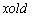, maximum number of  iterations as n, and error   tolerance as tol. Output the value of the root , number of iterations taken and the value of  f ( root ). If iterations n is not sufficient, the program should indicate the same. Assume that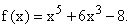(8)

Q.6     a.   A physicist wants to fit an approximation of the form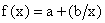to a data. Derive the normal equations using least squares approximation. Fit the above approximation to the following data.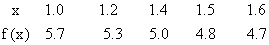(8)

b.   Construct the backward difference table for the data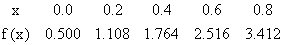Hence, compute an approximation to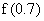.                                                           (8)

Q.7     a.   A differentiation formula is defined as follows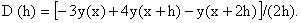Using the Taylor series expansions, show that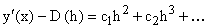and write   the expression for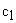. Use the above formula to compute approximations to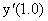with step lengths      h = 0.2 and h = 0.1 from the following table of values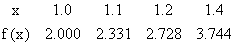(8)

b.   The error in composite Simpson’s rule with step length h for computing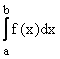is bounded by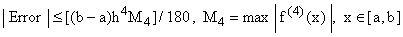. Assume that the composite Simpson’s rule is being used to compute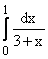. Using the above error estimate find h such that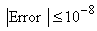.                                                           (8)

Q.8     a.   Derive the two point Gauss-Laguerre formula of integration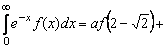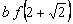. Hence, evaluate the integral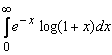.                (6+3)

b. Write a C program for solving an initial  value problem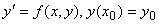using Euler’s method. Initial values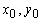, step length h and final value xf up to which the computations are to be carried out are to be read. Write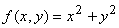as a function sub program. Output all the given data and all computed values.         (7)

Q.9     a.   Use Taylor series method of second order to compute y(1.4) with h = 0.2, for the initial value problem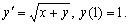(8)

b.   Error in Euler’s method for solving the initial value problem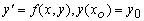is given by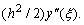Euler’s method is being used to solve the initial value problem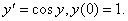Find the largest value of the step length h that can be used such that the magnitude of the error is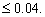(8)